Study Materials: ncert solutions

Our ncert solutions for Solutions 5. Continuity And Differentiability - Exercise 5.2 | Class 12 Mathematics-I - Toppers Study is the best material for English Medium students cbse board and other state boards students.

Solutions ⇒ Class 12th ⇒ Mathematics-I ⇒ 5. Continuity And Differentiability

# Solutions 5. Continuity And Differentiability - Exercise 5.2 | Class 12 Mathematics-I - Toppers Study

Topper Study classes prepares ncert solutions on practical base problems and comes out with the best result that helps the students and teachers as well as tutors and so many ecademic coaching classes that they need in practical life. Our ncert solutions for Solutions 5. Continuity And Differentiability - Exercise 5.2 | Class 12 Mathematics-I - Toppers Study is the best material for English Medium students cbse board and other state boards students.

## Solutions 5. Continuity And Differentiability - Exercise 5.2 | Class 12 Mathematics-I - Toppers Study

CBSE board students who preparing for class 12 ncert solutions maths and Mathematics-I solved exercise chapter 5. Continuity And Differentiability available and this helps in upcoming exams 2023-2024.

### You can Find Mathematics-I solution Class 12 Chapter 5. Continuity And Differentiability

• All Chapter review quick revision notes for chapter 5. Continuity And Differentiability Class 12
• NCERT Solutions And Textual questions Answers Class 12 Mathematics-I
• Extra NCERT Book questions Answers Class 12 Mathematics-I
• Importatnt key points with additional Assignment and questions bank solved.

Chapter 5 Mathematics-I class 12

### Exercise 5.2 class 12 Mathematics-I Chapter 5. Continuity And Differentiability

• Solutions 5. Continuity And Differentiability - Exercise 5.2 | Class 12 Mathematics-I - Toppers Study
• Class 12 Ncert Solutions
• Solution Chapter 5. Continuity And Differentiability Class 12
• Solutions Class 12
• Chapter 5. Continuity And Differentiability Exercise 5.2 Class 12

## Solutions 5. Continuity And Differentiability - Exercise 5.2 | Class 12 Mathematics-I - Toppers Study

Exercise - 5.2

Differentiate the functions with respect to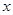in Exercise 1 to 8.

Ques.1.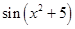Ans. Let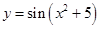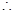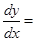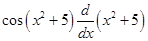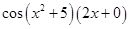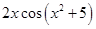Ques.2.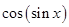Ans. Let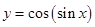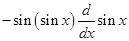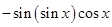Ques.3.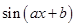Ans. Let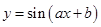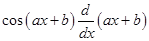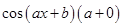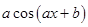Ques.4.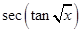Ans. Let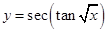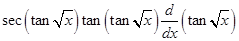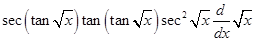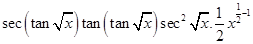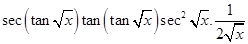Ques5.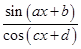Ans. Let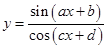Using quotient rule,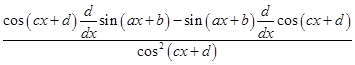=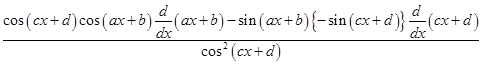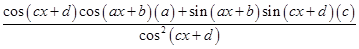Ques.6.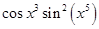Ans. Let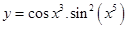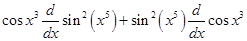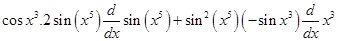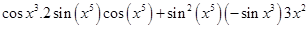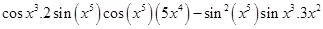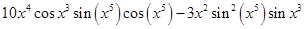Ques.7.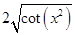Ans. Let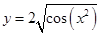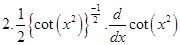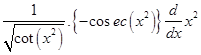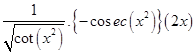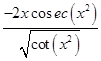Ques.8.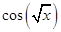Ans. Let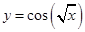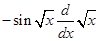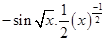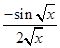### Ques.9. Prove that the function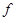given by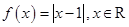is not differentiable at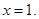Ans. Given: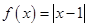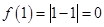R.H.L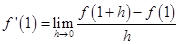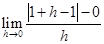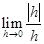=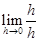= 1

And L.H.L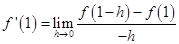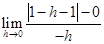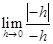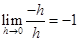Since   R.H.L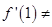L.H.L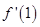hence,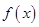is not differentiable at### Ques.10. Prove that the greatest integer function defined by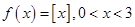is not differentiable at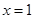and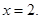Ans. Given:R.H.L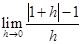=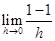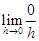= 0

And L.H.L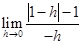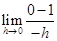=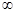Since   R.H.LL.H.Lhance,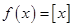is not differentiable atSimilarly,is not differentiable atImportant Study materials for classes 06, 07, 08,09,10, 11 and 12. Like CBSE Notes, Notes for Science, Notes for maths, Notes for Social Science, Notes for Accountancy, Notes for Economics, Notes for political Science, Noes for History, Notes For Bussiness Study, Physical Educations, Sample Papers, Test Papers, Mock Test Papers, Support Materials and Books.Mathematics Class - 11th

NCERT Maths book for CBSE Students.

books

## Study Materials List:

##### Solutions ⇒ Class 12th ⇒ Mathematics-I
1. Relations and Functions
2. Inverse Trigonometric Functions
3. Matrices
4. Determinants
5. Continuity And Differentiability
6. Application of Derivatives

New Books